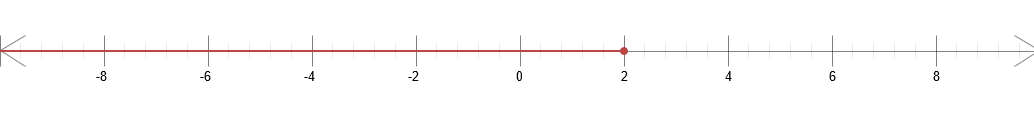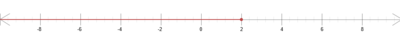Chapter 1 - Section 1.8 - Inequalities - 1.8 Exercises - Page 89: 17

($-\infty, 2]$$Solve$ $the$ $linear$ $inequality$. $Express$ $the$ $solution$ $using$ $interval$ $notation$ $and$ $graph$ $the$ $solution$ $set$: $7-x \geq 5$ Solve for x $7-x \geq 5$ Subtract 7 from both sides $7-7-x \geq 5-7$ $-x \geq -2$ Divide both sides by -1 (Switch the sign as well) $\frac{-x}{-1} \geq \frac{-2}{-1}$ $x \le 2$ Express the solution in interval notation form ($-\infty, 2]$ Graph the solution set